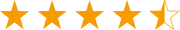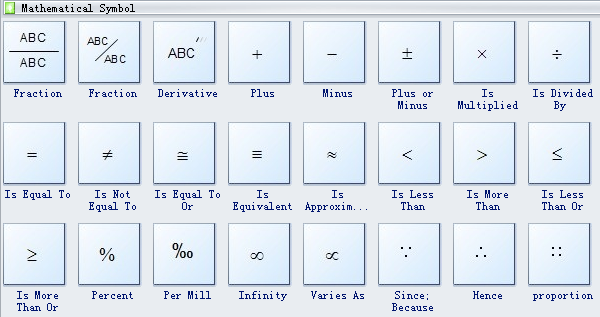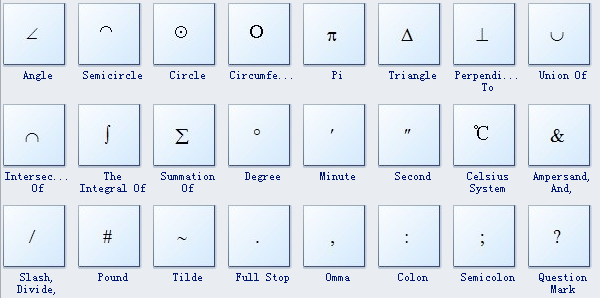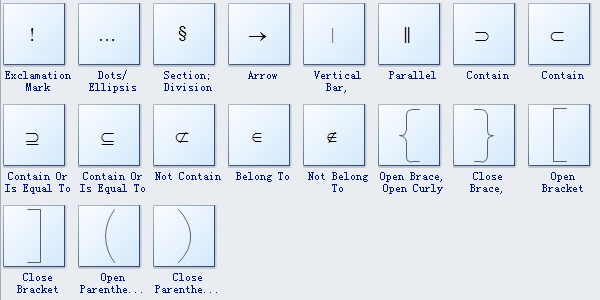A versatile cross-platform mind mapping tool.Get from App StoreA versatile cross-platform mind mapping tool.Get from App Store
edraw-max-horizontal
Product Tour
Templates
Guide
What's New
EdrawMax Online
TRY IT FREE

# Mathematics Diagram Symbols

> Basic Diagram Symbols > Mathematics Diagram Symbols
Posted by James Freeman | 12/23/2019
Pre-drawn mathematics diagram symbols represent fraction, derivative, plus, minus, plus or minus, etc. These symbols help create accurate diagrams and documentation.

There are many mathematical symbols in Edraw software, fraction, derivative, plus, minus, plus or minus, is multiplied, is divided by, is equal to, is not equal to, is equal to or, is equivalent to, is approximately equal to, is less than, is more than, etc.

### Mathematics Diagram Symbols

Mathematics Diagram ShapesMathematics diagram shapes like fraction, derivative, plus, minus, plus or minus, is multiplied, is divided by, is equal to, is not equal to, is equal to or, is equivalent to, is approximately equal to are available here.

Fraction is a way of representing division of a 'whole' into 'parts'.

Percent is one part in every hundred.

Infinity is a number greater than any assignable quantity or countable number (symbols).Question mark is indicating a question.There are two types of square brackets: the open ([) and close (]) bracket. The open bracket has an open end towards the right, and the close bracket has an open end towards the left.

Need fresh looking mathematics diagram symbols for your design? These mathematics diagram symbols are a cinch to pop in. And their crisp, fine detail will make spectacular, easy-to-understand diagrams and presentations to your customers.

## Get Started! You Will Love This Easy-To-Use Diagram Software

EdrawMax is an advanced all-in-one diagramming tool for creating professional flowcharts, org charts, mind maps, network diagrams, UML diagrams, floor plans, electrical diagrams, science illustrations, and more. Just try it, you will love it!